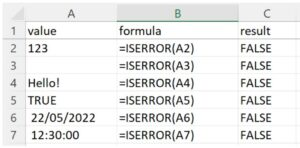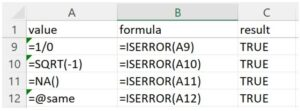## What is ISERROR?

ISERROR function is a logical function that is used to identify whether cells contain an error value or not. If there is any type of error in the cell it will return TRUE as result, and if not it returns FALSE.

Spreadsheet may contain a large quantity of data and formulas. Errors occur very often when building a spreadsheet. This will have an impact on the analysis if calculations are carried out using cells that contain errors. Sometimes the spreadsheet may not work properly and you won’t get a message telling you what is wrong. The ISERROR function can check for errors in a formula or in a macro and return a true or false value in any formula in Excel. It helps you debug so that the worksheet functions properly.

In order to keep the worksheets accurate and functioning properly without involving manual intervention, checking errors using the ISERROR function and preventing them is a key step.

Syntax:

=ISERROR(value)

## Key Learning Points

• Purpose of the function: The purpose is to test for any error and assist in further calculation.
• Parameters: Any variable or cell reference can be checked for an error, such as a number, text, mathematical operation, or expression. ISERROR can test both a single cell or a range of cells.
• Error codes: ISRROR tests most kinds of error messages. Below is a table of error codes that indicate different type of errors.
• Return value: The return result will be a logical expression. If the value or cell reference gives any of the errors above, the ISERROR function will return the result “TRUE”; conversely, it will return a “FALSE” result.
 Error type Meaning #N/A The function could not find the value it was asked to look for. Commonly occurs in VLOOKUP and similar functions. #VALUE! The given argument’s data type is incorrect or invalid. For example, the function =DATE(A1:A3) will return a #VALUE error when there is a text value instead of a number in the range. #REF! The given cell reference is incorrect or invalid. This error may occur when a cell/range has been deleted, moved, or pasted over. #DIV/0! The given cell is invalid. This occurs when a number is divided by zero (0), for example, a simple formula like =5/0, or when a formula refers to a cell that has 0 or is blank. #NUM! The given cell is invalid. It occurs when a calculation can’t be performed. For example, if you try to calculate the square root of a negative number. #NAME? The function name is incorrect or invalid. The given defined name (if any) is invalid. There is a typo in the defined name used in the function. Double quotes are missing for text values in the function. Colon missing in a cell range reference. #NULL! This means the cell contains nothing or is blank. This error occurs when there is something important missing with the range that can’t be identified in the formula you have supplied to the cell and is recognized in Excel by showing the error.

## More Detail

The ISERROR function can check which cell contains an error. If the cell contains an error then ISERROR returns TRUE, and if not it returns FALSE.

The ISERROR function is often used in conjunction with the IF function to test for errors and display a custom message or execute further actions when an error occurs.

## Examples

The ISERROR function is a basic function to check whether the input in cells has an error or not.The values in the example above are all correct, therefore the return result for each is shown as FALSE.Taking Cell A9 as an example, number 1 is divided by zero, which is an incorrect calculation. To check if the formula in the cell is valid or not, the syntax could just be as:

=ISERROR(1/0)

Alternatively we can refer to the cell reference as the example shown in the table above, using =ISERROR(A9). The return result is TRUE as it is an incorrect calculation.

## Conclusion

The ISERROR function simply returns a TRUE or FALSE if the cell contains or does not contain an error. Once you have the TRUE or FALSE, you can tell Excel what to do in each case using an IF function.